The OpenFOAM Foundationcomplex Class Reference

Extension to the c++ complex library type. More...

Collaboration diagram for complex: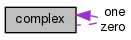[legend]

Public Types

typedef complex cmptType
Component type. More...

Public Member Functions

complex ()
Construct null. More...

complex (const scalar Re, const scalar Im)
Construct given real and imaginary parts. More...

complex (Istream &)
Construct from Istream. More...

scalar Re () const

scalar Im () const

scalar & Re ()

scalar & Im ()

complex conjugate () const

void operator+= (const complex &)

void operator-= (const complex &)

void operator*= (const complex &)

void operator/= (const complex &)

void operator= (const scalar)

void operator+= (const scalar)

void operator-= (const scalar)

void operator*= (const scalar)

void operator/= (const scalar)

complex operator! () const

bool operator== (const complex &) const

bool operator!= (const complex &) const

Static Public Attributes

static const char *const typeName = "complex"

static const complex zero

static const complex one

Friends

scalar magSqr (const complex &c)

complex sqr (const complex &c)

scalar mag (const complex &c)

const complexmax (const complex &, const complex &)

const complexmin (const complex &, const complex &)

complex limit (const complex &, const complex &)

const complexsum (const complex &)

complex operator+ (const complex &, const complex &)

complex operator- (const complex &)

complex operator- (const complex &, const complex &)

complex operator* (const complex &, const complex &)

complex operator/ (const complex &, const complex &)

complex operator* (const scalar, const complex &)

complex operator* (const complex &, const scalar)

complex operator/ (const complex &, const scalar)

complex operator/ (const scalar, const complex &)

Istreamoperator>> (Istream &, complex &)

Ostreamoperator<< (Ostream &, const complex &)

Detailed Description

Extension to the c++ complex library type.

Source files

Definition at line 76 of file complex.H.

◆ cmptType

 typedef complex cmptType

Component type.

Definition at line 86 of file complex.H.

◆ complex() [1/3]

 complex ( )
inline

Construct null.

Definition at line 33 of file complexI.H.

Here is the caller graph for this function: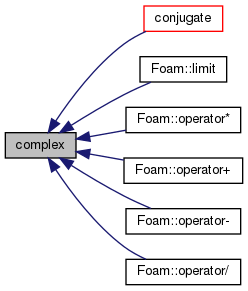◆ complex() [2/3]

 complex ( const scalar Re, const scalar Im )
inline

Construct given real and imaginary parts.

Definition at line 37 of file complexI.H.

◆ complex() [3/3]

 complex ( Istream & is )

Construct from Istream.

Definition at line 39 of file complex.C.

◆ Re() [1/2]

 scalar Re ( ) const
inline

Definition at line 46 of file complexI.H.

Referenced by Foam::name().

Here is the caller graph for this function: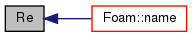◆ Im() [1/2]

 scalar Im ( ) const
inline

Definition at line 52 of file complexI.H.

Referenced by Foam::name().

Here is the caller graph for this function: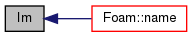◆ Re() [2/2]

 scalar & Re ( )
inline

Definition at line 58 of file complexI.H.

◆ Im() [2/2]

 scalar & Im ( )
inline

Definition at line 64 of file complexI.H.

◆ conjugate()

 complex conjugate ( ) const
inline

Definition at line 70 of file complexI.H.

References complex::complex().

Referenced by complex::operator!().

Here is the call graph for this function: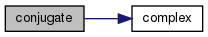Here is the caller graph for this function: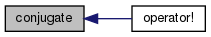◆ operator+=() [1/2]

 void operator+= ( const complex & c )
inline

Definition at line 78 of file complexI.H.

◆ operator-=() [1/2]

 void operator-= ( const complex & c )
inline

Definition at line 85 of file complexI.H.

◆ operator*=() [1/2]

 void operator*= ( const complex & c )
inline

Definition at line 92 of file complexI.H.

References Foam::constant::universal::c.

◆ operator/=() [1/2]

 void operator/= ( const complex & c )
inline

Definition at line 98 of file complexI.H.

References Foam::constant::universal::c.

◆ operator=()

 void operator= ( const scalar s )
inline

Definition at line 104 of file complexI.H.

References s().

Here is the call graph for this function: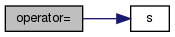◆ operator+=() [2/2]

 void operator+= ( const scalar s )
inline

Definition at line 111 of file complexI.H.

References s().

Here is the call graph for this function: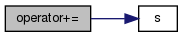◆ operator-=() [2/2]

 void operator-= ( const scalar s )
inline

Definition at line 117 of file complexI.H.

References s().

Here is the call graph for this function: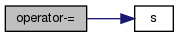◆ operator*=() [2/2]

 void operator*= ( const scalar s )
inline

Definition at line 123 of file complexI.H.

References s().

Here is the call graph for this function: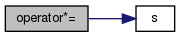◆ operator/=() [2/2]

 void operator/= ( const scalar s )
inline

Definition at line 130 of file complexI.H.

References s().

Here is the call graph for this function: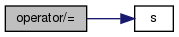◆ operator!()

 complex operator! ( ) const
inline

Definition at line 137 of file complexI.H.

References complex::conjugate().

Here is the call graph for this function: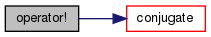◆ operator==()

 bool operator== ( const complex & c ) const
inline

Definition at line 143 of file complexI.H.

References Foam::equal().

Referenced by complex::operator!=().

Here is the call graph for this function: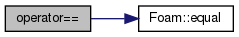Here is the caller graph for this function: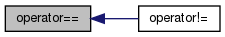◆ operator!=()

 bool operator!= ( const complex & c ) const
inline

Definition at line 149 of file complexI.H.

References complex::operator==().

Here is the call graph for this function: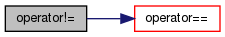◆ magSqr

 scalar magSqr ( const complex & c )
friend

Definition at line 158 of file complexI.H.

Referenced by Foam::mag(), and Foam::operator/().

◆ sqr

 complex sqr ( const complex & c )
friend

Definition at line 164 of file complexI.H.

◆ mag

 scalar mag ( const complex & c )
friend

Definition at line 170 of file complexI.H.

Referenced by Foam::max(), and Foam::min().

◆ max

 const complex& max ( const complex & c1, const complex & c2 )
friend

Definition at line 176 of file complexI.H.

◆ min

 const complex& min ( const complex & c1, const complex & c2 )
friend

Definition at line 189 of file complexI.H.

◆ limit

 complex limit ( const complex & c1, const complex & c2 )
friend

Definition at line 202 of file complexI.H.

Referenced by Foam::limit().

◆ sum

 const complex& sum ( const complex & c )
friend

Definition at line 208 of file complexI.H.

◆ operator+

 complex operator+ ( const complex & c1, const complex & c2 )
friend

Definition at line 225 of file complexI.H.

◆ operator- [1/2]

 complex operator- ( const complex & c )
friend

Definition at line 235 of file complexI.H.

◆ operator- [2/2]

 complex operator- ( const complex & c1, const complex & c2 )
friend

Definition at line 245 of file complexI.H.

◆ operator* [1/3]

 complex operator* ( const complex & c1, const complex & c2 )
friend

Definition at line 255 of file complexI.H.

◆ operator/ [1/3]

 complex operator/ ( const complex & c1, const complex & c2 )
friend

Definition at line 265 of file complexI.H.

◆ operator* [2/3]

 complex operator* ( const scalar s, const complex & c )
friend

Definition at line 277 of file complexI.H.

◆ operator* [3/3]

 complex operator* ( const complex & c, const scalar s )
friend

Definition at line 283 of file complexI.H.

◆ operator/ [2/3]

 complex operator/ ( const complex & c, const scalar s )
friend

Definition at line 289 of file complexI.H.

◆ operator/ [3/3]

 complex operator/ ( const scalar s, const complex & c )
friend

Definition at line 295 of file complexI.H.

◆ operator>>

 Istream& operator>> ( Istream & , complex & )
friend

◆ operator<<

 Ostream& operator<< ( Ostream & , const complex & )
friend

◆ typeName

 const char *const typeName = "complex"
static

Definition at line 91 of file complex.H.

◆ zero

 const Foam::complex zero
static

Definition at line 93 of file complex.H.

◆ one

 const Foam::complex one
static

Definition at line 94 of file complex.H.

The documentation for this class was generated from the following files: